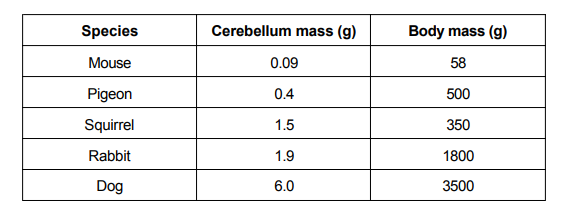# IMAT 2015 Q11 [Body Mass]

The table below shows the average mass of the cerebellum, which is a part of the brain, and total body mass, for a number of animal species. The cerebellum of a cat has an average mass of 5.3 g, while a cat’s average body mass is 3.5 kg.For which of the species shown is the ratio of cerebellum to total body mass closest to that of the cat?

A. Pigeon
B. Squirrel
C. Dog
D. Rabbit
E. Mouse

Simple steps to solve word problems:

• Underline key information
• Determine what they are trying to ask, and what you will need to solve it
• Eliminate any non-essential information
• Draw a picture, graph, or equation
• In moments of high stress like exam taking, always work with the paper they give you to avoid careless mistakes.
• Solve.

DO NOT BE AFRAID TO APPROXIMATE HERE!

This is not a hard question conceptually, but without a calculator, it can be difficult to tackle. Remember that we are working with ratios, so we can multiply or divide both sides of the ratios and it will still be a valid ratio.

First, let’s convert the cat’s mass to grams to make this easier:

3.5kg = 3500g

Brain : Body
5.3g : 3500g

Now, let’s take our options and try to get them to a similar range (in the low thousands). Let’s see what I mean by this:

Mouse

100 (0.09g) = (58g) 100

9g = 5800g

Now let’s find a closer relationship with the cat, lets do this by multiplying the mouse ratio by 2 and the cat ratio by 3

Mouse
2(9g) = 2(5800g)
18g = 11600g

Cat
3(5.3g) : 3(3500g)
15.9g : 10500g

Close, but let’s keep looking:

Pigeon

10 (0.4g) = (500g) 10
4g : 5000g

Compared to the cat, it is much too small, smaller brain size and a bigger body in this ratio. We can eliminate this option.

Squirrel

10 (1.5g) = (350g) 10
15g : 3500g

This ratio is much too big, we have the same weight but a much larger brain. Therefore we can eliminate this option.

Rabbit

2 (1.9g) = (1800g) 2
3.8g : 3600g

We can eliminate this option because it is not as close as the dog ratio is to the cat ratio.

Dog

6.0g = 3500g

This is extremely close.

We can narrow it down to between the mouse and dog.

Mouse
2(9g) = 2(5800g)
18g = 11600g

Cat
3(5.3g) : 3(3500g)
15.9g : 10500g

If we put the dog on the same scale:

Dog
6.0g = 3500g
3(6.0g = 3(3500g)
18g = 10500g

Notice how the cat and dog have the same body weight, and the mouse and the dog have the same brain weight at these ratios?

Therefore we can conclude that the mouse is more similar to the cat ratio. The mouse has a higher brain mass and a higher body mass than the cat when comparing these two ratios, so when it decreases, it will have a brain mass and a body mass which are smaller and more closer to the cat. The dog here already has the same body mass as the cat in this ratio, but it has the same brain size. This means that a mouse with a smaller ratio will have a smaller brain than the dog at a comparable size, which is closer to the brain size of the cat.

As a result, we can conclude that the answer is E, the mouse.

Proof using a calculator:

Take the ratio off all three animals:

Cat:

\frac{3500g}{5.3g} = 660.4

Dog:

\frac{3500g}{6g} = 583.4

Mouse:

\frac{58g}{0.09g} = 644,4

Cat > Mouse > Dog

Mouse and cat have the closest cerebellum to body mass ratio.

1 Like